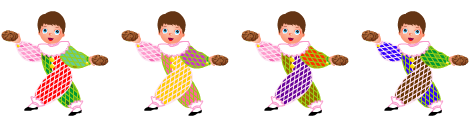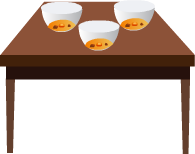Upgrade to remove ads
Upgrade to remove ads
Upgrade to remove ads
13,231 Reads

# Multiplying Fractions with Whole Numbers

Rate Lesson 0 stars
Lesson size:
Message preview:
Someone you know has shared lesson with you:

To play this lesson, click on the link below:

https://www.turtlediary.com/lesson/multiplying-fractions-with-whole-numbers.html

To know more about different lessons, please visit www.turtlediary.com

Hope you have a good experience with this site and recommend to your friends too.

Login to rate activities and track progress.
Login to rate activities and track progress.

Multiplying fractions with whole numbers is much easier than adding fractions.

Let us learn this by following examples :

## Example 1

If we have four children and each has two cookies, how many cookies are there in all?If we multiply 4 x 2, we find that there are 8 cookies total.

But what if we have four children and each has
2
3
of a cookie? How many cookies are there in all?

Just like with whole numbers, we can use multiplication to solve a problem like this.

Multiply the numerator by the whole number, and leave the denominator the same.

We need to multiply 4 x
2
3
First, multiply the numerator by the whole number.

That's your new numerator!

4 x 2 = 8

Remember, the denominator stays the same, so our answer is:

8
3

Since the numerator is larger than the denominator, you can tell that this fraction is bigger than one.

You can restate it as 2
2
3
.

Here is how we did it. To change an improper fraction to a mixed number, you must divide the numerator by the denominator. Then, the mixed number takes the following form :

• The answer becomes the whole number
• The remainder becomes the numerator
• The denominator stays the same## Example 2

There are three bowls of soup on a table. Each bowl is
1
5
full. How much soup is there in total?We need to multiply 3 x
1
5
.

3 x 1
5
=
3
5

There is
3
5
of a bowl soup in total.
Summary
• Fractions represent parts of whole numbers
• Numerator (number on top) represents how many parts of the whole you have
• Denominator (number on the bottom) represents how many equal parts the whole is divided into.
• Multiplying Fractions by a Whole Number: Multiply the numerator by the whole number, and leave the denominator the same.

## More Fractions Games

Become premium member to get unlimited access.• •  Unlimited access to over thousands of worksheets and activities for all grade levels.
• •  Award-winning educational games and videos.
• •  Teacher created quizzes with step by step solution.
• •  Ad-free experience for children.
• •  Unlimited access to Interactive Stories with "Read to me" feature.
• •  Informative assessment tools with detailed reports pointing out successes and weak spots.
• •  Audio Instructions for all games.